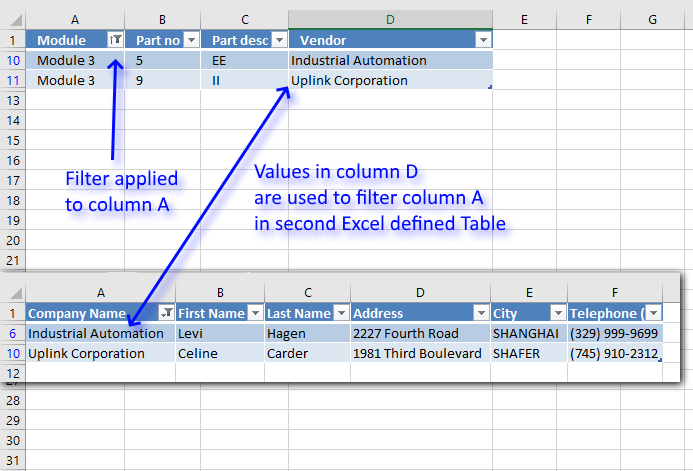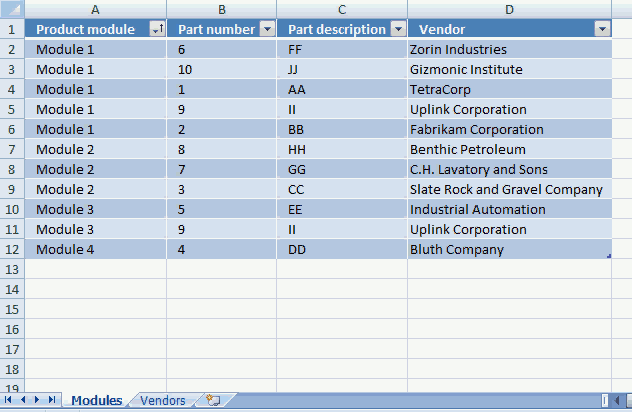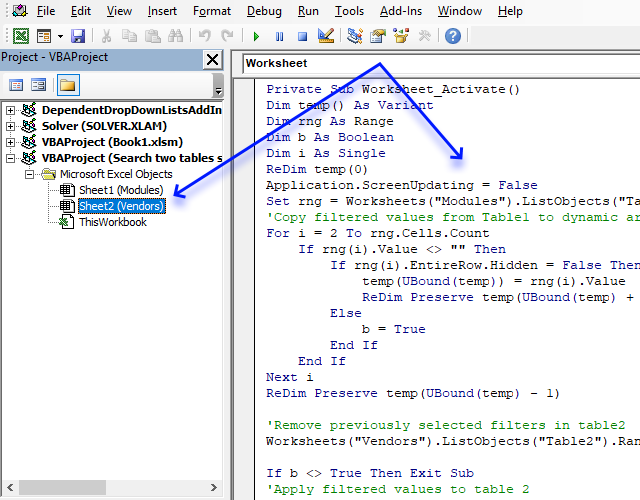Author: Oscar Cronquist Article last updated on August 27, 2019This article demonstrates a macro that automatically applies a filter to an Excel defined Table based on the result from a second Excel defined Table.

Let's say you do a lot of searches in two tables. The tables are related so it would be great if the second table is simultaneously filtered depending on the filtered values from the first table.

Two data sets are related if they share at least one column, that makes it possible to perform two searches or lookups.Example,

You want to know the contact information to all vendors in product module 3. You select Module 3 in column "Product module" and vendor names appear in column "Vendor".

But contact information to each vendor is in table 2, sheet "Vendors". The macro demonstrated in the animated picture below filters table2 automatically.

The following event code is rund when worksheet "Vendors" is activated.

```'Event code that runs when worksheet is activated (selected)
Private Sub Worksheet_Activate()

'Dimension variables and declare data types
Dim temp() As Variant
Dim rng As Range
Dim b As Boolean
Dim i As Single

'Redimension variable temp to make it possible to add more values later on in this macro
ReDim temp(0)

'Don't show changes on screen
Application.ScreenUpdating = False

'Save the fourth column in Table1 to object variable rng
Set rng = Worksheets("Modules").ListObjects("Table1").ListColumns(4).Range

'Copy filtered values from Table1 to growing array

'Iterate through cells in cell range except header cell
For i = 2 To rng.Cells.Count

'Check if value is not equal to nothing
If rng(i).Value <> "" Then

'Check that row is not filtered out
If rng(i).EntireRow.Hidden = False Then

'Save value to array variable temp
temp(UBound(temp)) = rng(i).Value

'Increase size of array variable temp
ReDim Preserve temp(UBound(temp) + 1)
Else

'Save boolean value True to variable b, this will apply a filter to the other Excel defined Table later on in this event code
b = True
End If
End If
Next i

'Remove last container from array variable temp
ReDim Preserve temp(UBound(temp) - 1)

'Remove previously selected filters in table2
Worksheets("Vendors").ListObjects("Table2").Range.AutoFilter Field:=1

'Check if variable b is False and stop this macro if so
If b <> True Then Exit Sub

'Apply filtered values to table 2
Worksheets("Vendors").ListObjects("Table2").Range.AutoFilter _
Field:=1, Criteria1:=temp, Operator:=xlFilterValues

'Show changes to Excel user
Application.ScreenUpdating = True
End Sub
```

### Where to put the code?1. Copy above event code.
2. Press Alt + F11 to open the Visual Basic Editor.
3. Doublepress with left mouse button on a worksheet in your workbook to open the worksheet module.
4. Paste code to worksheet module.

### Get the Excel fileSearch-two-tables-simultaneously.xlsm